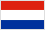Dipl. Ing. Univerzitet u Beogradu 1995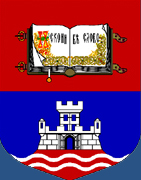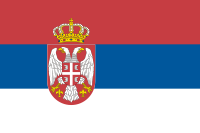Ph.D. University of Illinois at Chicago 2001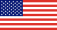B.S., M.S., Technion 1976, 1979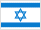Ph.D. Stanford University 1983Dipl. Ing. Eidgenössische Technische Hochschule Zürich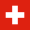Ph.D. Stanford University 1974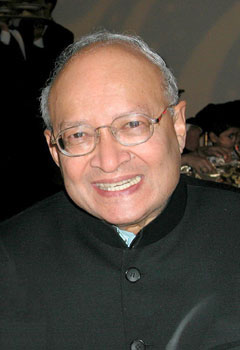B.E. Poone 1956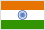Sc.D. Massachusetts Institute of Technology 1961Kailath's Sc.D. Adviser:           Sc.D. Massachusetts Institute of Technology 1957Wozencraft's Sc.D. Adviser:           studied at Politecnico di Torino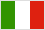B.S., Sc.D. Massachusetts Institute of Technology 1941, 1947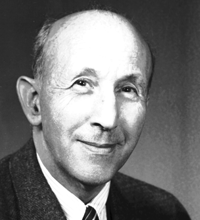B.S. University of Wisconsin 1922Ph.D. Ludwig-Maximilians-Universität München 1926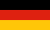"We refer to things being advanced only so long as we understand them insufficiently ourselves to be able to make them clear in simple terms."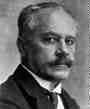Guillemin's Adviser:         Arnold Johannes Wilhelm Sommerfeld

Ph.D. Universität Königsberg 1891Arnold Sommerfeld pioneered developments in atomic and quantum physics, as well as educated and groomed a large number of students for the new era of theoretical physics.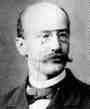Sommerfeld's Adviser:         Carl Louis Ferdinand von Lindemann

Ph.D. Friedrich-Alexander-Universität Erlangen-Nürnberg 1873Ferdinand von Lindemann was the first to prove that π is transcendental, i.e. that π is not a root of any algebraic equation with rational coefficients.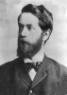Lindemann's Adviser:  Felix Christian Klein

( > 35000 descendants)

Ph.D. Rheinische Friedrich-Wilhelms-Universität Bonn 1868Felix Klein is best known for his work in non- euclidean geometry, for his work on the connections between geometry and group theory, and for results in function theory. However Klein considered his work in function theory to be the summit of his work in mathematics. He owed some of his greatest successes to his development of Riemann's ideas and to the intimate alliance he forged between the later and the conception of invariant theory, of number theory and algebra, of group theory, and of multidimensional geometry and the theory of differential equations, especially in his own fields, elliptic modular functions and automorphic functions.

Klein's Adviser 1: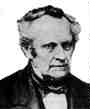Ph.D. Philipps-Universität Marburg 1823Julius Plücker was educated at Heidelberg, Berlin and Paris. He made important contributions to analytic geometry and physics.

Christian Ludwig Gerling

Ph.D. Georg-August-Universität Göttingen 1812Dissertation: Methodi proiectionis orthographicae usum ad calculos parallacticos facilitandos explicavit simulque eclipsin solarem die
He was a student of the mathematician Johann Carl Friedrich Gauß. Since 1817 he worked as an astronomer in Marburg.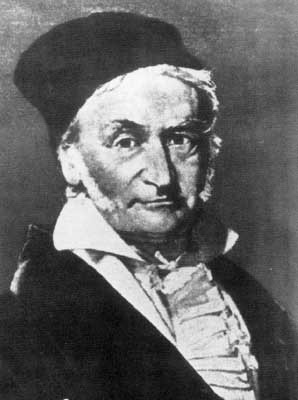Johann Carl Friedrich Gauß
( > 50000 descendants)
Ph.D. Universität Helmstedt 1799At the age of seven, Carl Friedrich Gauß started elementary school, and his potential was noticed almost immediately. His teacher, Büttner, and his assistant, Martin Bartels, were amazed when Gauss summed the integers from 1 to 100 instantly by spotting that the sum was 50 pairs of numbers each pair summing to 101.
In 1798, he had made one of his most important discoveries - the construction of a regular 17-gon by ruler and compasses This was the most major advance in this field since the time of Greek mathematics and was published as Section VII of Gauss's famous work, Disquisitiones Arithmeticae. He published his second book, Theoria motus corporum coelestium in sectionibus conicis Solem ambientium, in 1809, a major two volume treatise on the motion of celestial bodies. In the first volume he discussed differential equations, conic sections and elliptic orbits, while in the second volume, the main part of the work, he showed how to estimate and then to refine the estimation of a planet's orbit.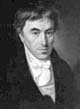Johann Friedrich Pfaff
Ph.D. Georg-August-Universität Göttingen 1786Pfaff's inaugural dissertation was titled Programma inaugurale in quo peculiarem differentialia investigandi rationem ex theoria functionum deducit. It investigates the use of some functional equations in order to calculate the differentials of logarithmic and trigonometrical functions as well as the binomial expansion and Taylor formula. Pfaff did important work in analysis working on partial differential equations, special functions and the theory of series. He developed Taylor's Theorem using the form with remainder as given by Lagrange. In 1810 he contributed to the solution of a problem due to Gauss concerning the ellipse of greatest area which could be drawn inside a given quadrilateral.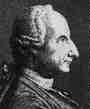Abraham Gotthelf Kästner

Ph.D. Universität Leipzig 1739Perhaps the most important feature of Kästner's contributions was his interest in the parallel postulate which indirectly influenced Bolyai and Lobachevsky too. Kästner taught Bolyai's father and J M C Bartels, one of Kästner's students, taught Lobachevsky.

Johann Elert Bode
Ph.D. Handelsakademie HamburgChristian August Hausen
Ph.D. Martin-Luther-Universität Halle-Wittenberg 1713Johann Christoph Wichmannshausen
Ph.D. Universität Leipzig 1685Johann Andreas Planer
Medicinae Dr. Eberhard-Karls-Universität Tübingen 1709Johann Pasch
Magister Artium Martin-Luther-Universität Halle-Wittenberg 1683Rudolf Jakob Camerarius
Medicinae Dr. Eberhard-Karls-Universität Tübingen 1684, 1686Georg Balthasar Metzger
Medicinae Dr. Friedrich-Schiller-Universität Jena 1644Universität Basel 1650Metzger's Adviser 1: Johann Georg Macasius Medicinae Dr. Friedrich-Schiller-Universität Jena 1638, 1640Macasius' Adviser 1: Johannes Musaeus Magister Artium Universität Erfurt 1634Musaeus' Adviser: Georg Großhain Magister Artium Martin-Luther-Universität Halle-Wittenberg 1629Metzger's Adviser 2: Emmanuel Stupanus Medicinae Dr. Universität Basel 1613Stupanus' Adviser: Petrus Ryff Medicinae Dr. Universität Basel 1584Ryff's Adviser: Theodor Zwinger Magister Artium Collège de France 1553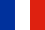Zwinger's Adviser: Peter Ramus Artium Liberalium Magister Collège de Navarre 1536Ramus' Adviser 1: Johann Sturm Magister Artium Université Catholique de Louvain 1527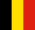Sturm's Adviser 1: Nicolas Clénard Magister Artium, Theol. Dr. Université Catholique de Louvain 1515, 1521Sturm's Adviser 2: Johannes Winter von Andernach Magister Artium Université Catholique de Louvain 1527, Med. Dr. Collège de Tréguier 1532von Andernach's Adviser 1: Rutger Rescius Artium Baccalaureus Université de Paris 1513Rescius' Adviser: Girolamo Aleandro Magister Artium, Theol. Dr. Università di Padova 1499, 1508Aleandro's Adviser: Scipione Fortiguerra Magister Artium Università di Firenze 1493Fortiguerra's Adviser: Angelo Poliziano Magister Artium Università di Firenze 1477Interestingly, Poliziano is an adviser of Michelangelo Buonarroti, Baccalaureus Artium Università di Firenze 1492. Poliziano's Adviser: Marsilio Ficino Magister Artium Università di Firenze 1462Ficino's Adviser: Johannes Argyropoulos Theol. Dr. Università di Padova 1444Interestingly, Argyropoulos is an adviser of Leonardo da Vinci, Baccalaureus Artium Università di Firenze 1471.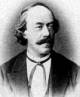Rudolf Otto Sigismund Lipschitz

Ph.D. Universität Berlin 1853Rudolf Lipschitz worked on quadratic differential forms and mechanics. Lipschitz rediscovered the Clifford algebras and was the first to apply them to represent rotations of Euclidean spaces, thus introducing the spin groups Spin(n).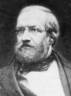Lipschitz's Adviser:

Ph.D. Rheinische Friedrich-Wilhelms-Universität Bonn (Hon.) 1827Dirichlet proved in 1826 that in any arithmetic progression with first term coprime to the difference there are infinitely many primes. Dirichlet is best known for his papers on conditions for the convergence of trigonometric series and the use of the series to represent arbitrary functions.

 Dirichlet's Adviser 1: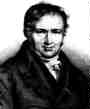Siméon-Denis Poisson ( > 60000 descendants) École PolytechniquePoisson published between 300 and 400 mathematical works including applications to electricity and magnetism, and astronomy. His Traité de mécanique published in 1811 and again in 1833 was the standard work on mechanics for many years.  His name is attached to a wide area of ideas, for example:- Poisson's  distribution, Poisson's integral, Poisson's equation in potential theory, Poisson brackets in differential equations, Poisson's ratio in elasticity, and Poisson's constant in electricity. Dirichlet's Adviser 2: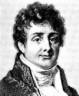Jean-Baptiste Joseph Fourier École PolytechniqueFourier studied the mathematical theory of heat conduction. He established the partial differential equation governing heat diffusion and solved it by using infinite series of trigonometric functions.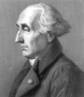Poisson's and Fourier's Adviser:(or, perhaps, Giuseppe Lodovico Lagrangia)

Lagrange excelled in many topics: astronomy, the stability of the solar system, mechanics, dynamics, fluid mechanics, probability, and the foundations of the calculus. He also worked on number theory proving in 1770 that every positive integer is the sum of four squares. In 1771 he proved Wilson's theorem (John Wilson) (first stated without proof by Waring) that n is prime if and only if (n-1)!+1 is divisible by n. In 1770 he also presented his important work Réflexions sur la résolution algébrique des équations which made a fundamental investigation of why equations of degrees up to 4 could be solved by radicals. The paper is the first to consider the roots of a equation as abstract quantities rather than having numerical values. He studied permutations of the roots and, although he does not compose permutations in the paper, it can be considered as a first step in the development of group theory continued by Ruffini, Galois and Cauchy.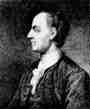Leonhard Euler
Ph.D. Universität Basel 1726Euler was the most prolific writer of mathematics of all time.
He made decisive and formative contributions to geometry, calculus and number theory. He integrated Leibniz's differential calculus and Newton's method of fluxions into mathematical analysis. He introduced beta and gamma functions, and integrating factors for differential equations. He studied continuum mechanics, lunar theory with Clairaut, the three body problem, elasticity, acoustics, the wave theory of light, hydraulics, and music. He laid the foundation of analytical mechanics, especially in his Theory of the Motions of Rigid Bodies (1765).
We owe to Euler the notation f(x) for a function (1734), e for the base of natural logs (1727), i for the square root of -1 (1777), p for pi,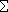for summation (1755), the notation for finite differences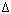y and2y and many others.
In 1737 he proved the connection of the zeta function with the series of prime numbers.
One could claim that mathematical analysis began with Euler. In 1748 in Introductio in analysin infinitorum Euler made ideas of Johann Bernoulli more precise in defining a function, and he stated that mathematical analysis was the study of functions. In Introductio in analysin infinitorum Euler dealt with logarithms. He published his full theory of logarithms of complex numbers in 1751.
The Mathematics Genealogy project lists Euler as Lagrange's adviser. Indeed, Euler did give substantial advice to Lagrange, although all of it was by mail. Lagrange studied Euler's work and corresponded extensively with him during the time when he wrote his early papers. Euler was also important in promoting Lagrange's career. This is why Euler is often viewed as his "adviser," even though Euler was in Berlin and Lagrange was in Torino.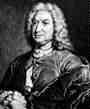Johann Bernoulli
1694Bernoulli was  de l'Hôpital's tutor. However it did assure de l'Hôpital of a place in the history of mathematics since he published the first calculus book Analyse des infiniment petits pour l'intelligence des lignes courbes (1696) which was based on the lessons that Johann Bernoulli sent to him (without acknowledging that fact). The well-known de l'Hôpital's rule is contained in this calculus book and it is therefore a result of Johann Bernoulli. Bernoulli also made important contributions to mechanics with his work on kinetic energy.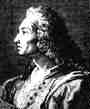Jacob Bernoulli
1676Jacob Bernoulli's first important contributions were a pamphlet on the parallels of logic and algebra published in 1685, work on probability in 1685 and geometry in 1687. His geometry result gave a construction to divide any triangle into four equal parts with two perpendicular lines.
By 1689 he had published important work on infinite series and published his law of large numbers in probability theory. The interpretation of probability as relative-frequency says that if an experiment is repeated a large number of times then the relative frequency with which an event occurs equals the probability of the event. The law of large numbers is a mathematical interpretation of this result. Jacob Bernoulli published five treatises on infinite series between 1682 and 1704. The first two of these contained many results, such as fundamental result that(1/n) diverges, which Bernoulli believed were new but they had actually been proved by Mengoli 40 years earlier. Bernoulli could not find a closed form for(1/n2) but he did show that it converged to a finite limit less than 2. Euler was the first to find the sum of this series in 1737. Bernoulli also studied the exponential series which came out of examining compound interest.
In May 1690 in a paper published in Acta Eruditorum, Jacob Bernoulli showed that the problem of determining the isochrone is equivalent to solving a first-order nonlinear differential equation.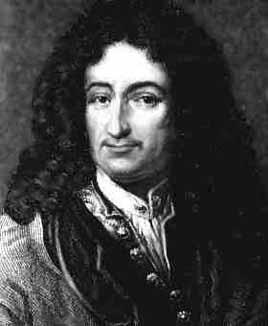Jacob Bernoulli's Adviser:         Gottfried Wilhelm von Leibniz

Dr. jur. Universität Altdorf 1667Gottfried Leibniz developed the present-day notation for the differential and integral calculus though he never thought of the derivative as a limit. His philosophy is also important and he invented an early calculating machine.

Ph.D. Universität Leipzig 1650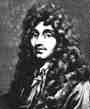Universiteit Leiden